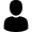Capgemini First round is aptitude round -containing 16 questions from quants and 16 from logical reasoning. Duration -1 hour Quants have more weightage from Time speed distance. Around 5 questions asked from that topic. 2nd round is pseudocode round. Where you'll be given 20 questions to solve in20 minutes. Questions are asked from data structure and some basic algorithms are given to solve. Third round is Techinal HR round Where they asked basic questions of programming language and some questions related to your resume and the final round is HR round.

# First round is aptitude round -containing 16 questions from quants and 16 from logical reasoning. Duration -1 hour Quants have more weightage from Time speed distance. Around 5 questions asked from that topic. 2nd round is pseudocode round. Where you'll be given 20 questions to solve in20 minutes. Questions are asked from data structure and some basic algorithms are given to solve. Third round is Techinal HR round Where they asked basic questions of programming language and some questions related to your resume and the final round is HR round.

CapgeminiVijay sunder S
0 2 103
2 years ago

Capgemini Interview Experience

First round is aptitude round -containing 16 questions from quants and 16 from logical reasoning. Duration -1 hour Quants have more weightage from Time speed distance. Around 5 questions asked from that topic. 2nd round is pseudocode round. Where you'll be given 20 questions to solve in20 minutes. Questions are asked from data structure and some basic algorithms are given to solve. Third round is Techinal HR round Where they asked basic questions of programming language and some questions related to your resume and the final round is HR round.